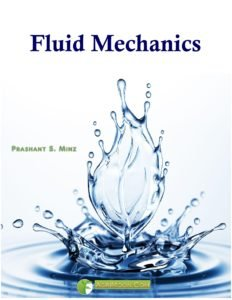You are here

Book Detail: Fluid MechanicsLanguage: English

Pages: 179

Author: Prashant S. Minz

Price: Free

Course Outlines: Fluid Mechanics 3(2+1)

Module 1: Introduction to fluid mechanics
Lesson 1. Units and dimensions, Properties of fluids.

Module 2 : Static pressure of liquids
Lesson 2. Static pressure of liquids: Hydraulic pressure, absolute and gauge pressure
Lesson 3. Pressure head of a liquid, Pressure on vertical rectangular surfaces
Lesson 4. Compressible and non compressible fluids. Surface tension, capillarity.

Module 3: Pressure measuring devices
Lesson 5. Simple manometer, piezometer, U tube manometer
Lesson 6. Micro manometers and Inclined manometer
Lesson 7. Differential manometers
Lesson 8. Mechanical gauges

Module 4: Floating bodies
Lesson 9. Archimedes principle, stability of floating bodies
Lesson 10. Equilibrium of floating bodies. Metacentric height

Module 5: Fluid flow
Lesson 11. Classification, steady uniform and non uniform flow, Laminar and turbulent
Lesson 12. Continuity equation
Lesson 13. Bernoulli’s theorem
Lesson 14. Application of Bernoulli’s theorem

Module 6: Flow through pipes
Lesson 17. Problems on head loss
Lesson 18. Determination of pipe diameter, determination of discharge, friction factor, critical velocity.

Module 7: Flow through orifices, mouthpieces, notches and weirs
Lesson 19. Orifices, vena contracta, Hydraulic coefficients
Lesson 20. Discharge losses, Time for emptying a tank
Lesson 21. External and internal Mouthpiece
Lesson 22. Types of notches, rectangular and triangular notches, rectangular weirs
Lesson 23. Numericals on orifice, mouthpiece, notch and weir

Module 8: Measuring Instruments
Lesson 24. Venturimeter and pitot tube
Lesson 25. Rotameter, Water level point gauge, hook gauge

Module 9: Dimensional analysis
Lesson 26. Buckingham’s theorem application to fluid flow phenomena
Lesson 27. Froude Number, Reynolds number, Weber number
Lesson 28. Hydraulic similitude

Module 10 : Pumps
Lesson 29. Classification of pumps
Lesson 30. Reciprocating pumps
Lesson 31. Centrifugal pump, Pressure variation, work done, efficiency
Lesson 32. Numericals on pumps

Disclaimer: The information on this website does not warrant or assume any legal liability or responsibility for the accuracy, completeness or usefulness of the courseware contents.

The contents are provided free for noncommercial purpose such as teaching, training, research, extension and self learning.

1.Akanksha Joshi says: